# Please do question 5a and 5b 4. In this problem we analyze the behavior of the...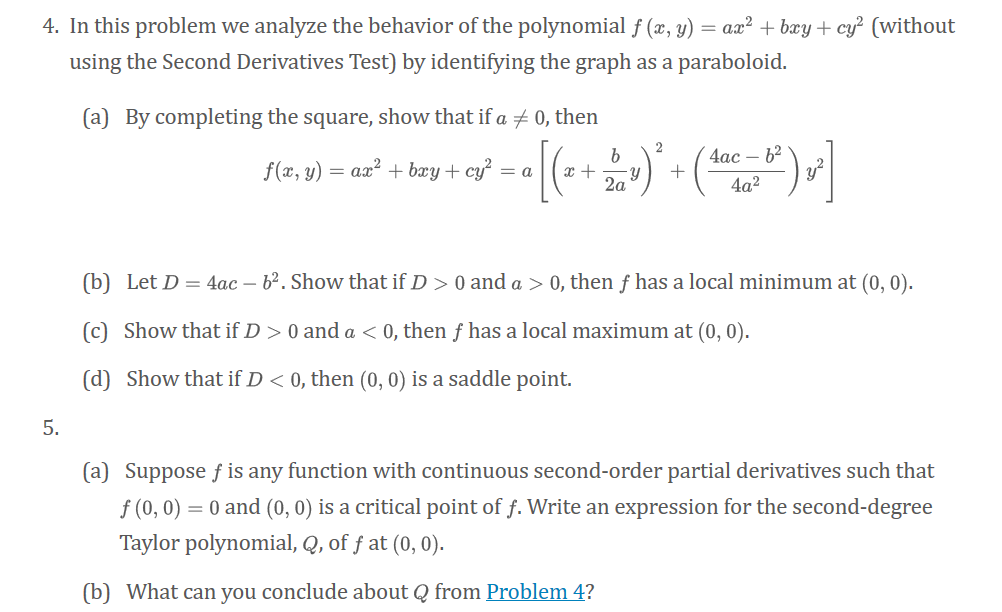Please do question 5a and 5b

4. In this problem we analyze the behavior of the polynomial f (x, y) = ax² + bxy + cy? (without using the Second Derivatives Test) by identifying the graph as a paraboloid. (a) By completing the square, show that if a + 0, then b 2 4ac - 62 f(x, y) = ax² + bxy + cy? = a [( 2 + Y + 2a 4a2 (b) Let D = 4ac – 62. Show that if D > 0 and a > 0, then f has a local minimum at (0,0). (C) Show that if D > 0 and a < 0, then f has a local maximum at (0,0). (d) Show that if D< 0, then (0,0) is a saddle point. 5. (a) Suppose f is any function with continuous second-order partial derivatives such that f(0,0) = 0 and (0,0) is a critical point of f. Write an expression for the second-degree Taylor polynomial, Q, of f at (0,0). (b) What can you conclude about Q from Problem 4?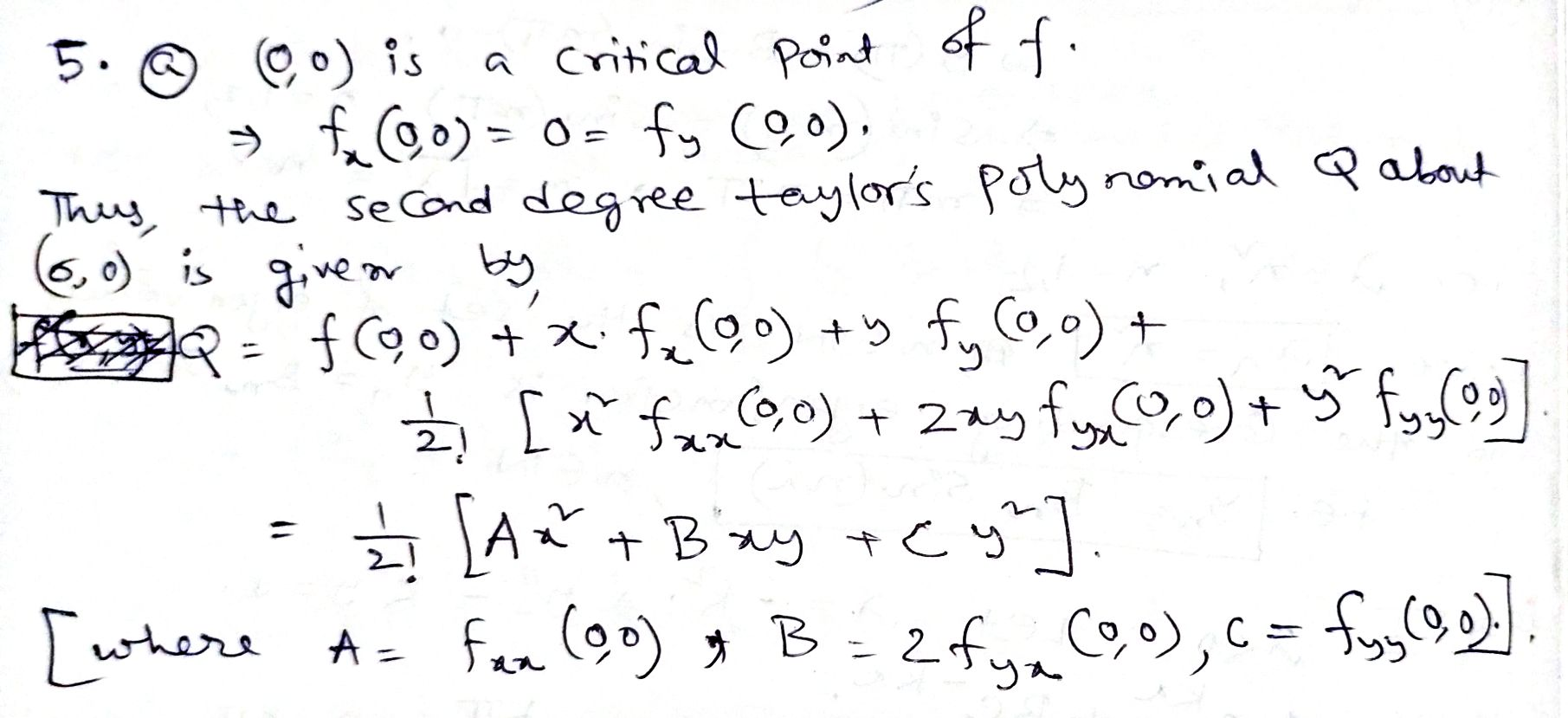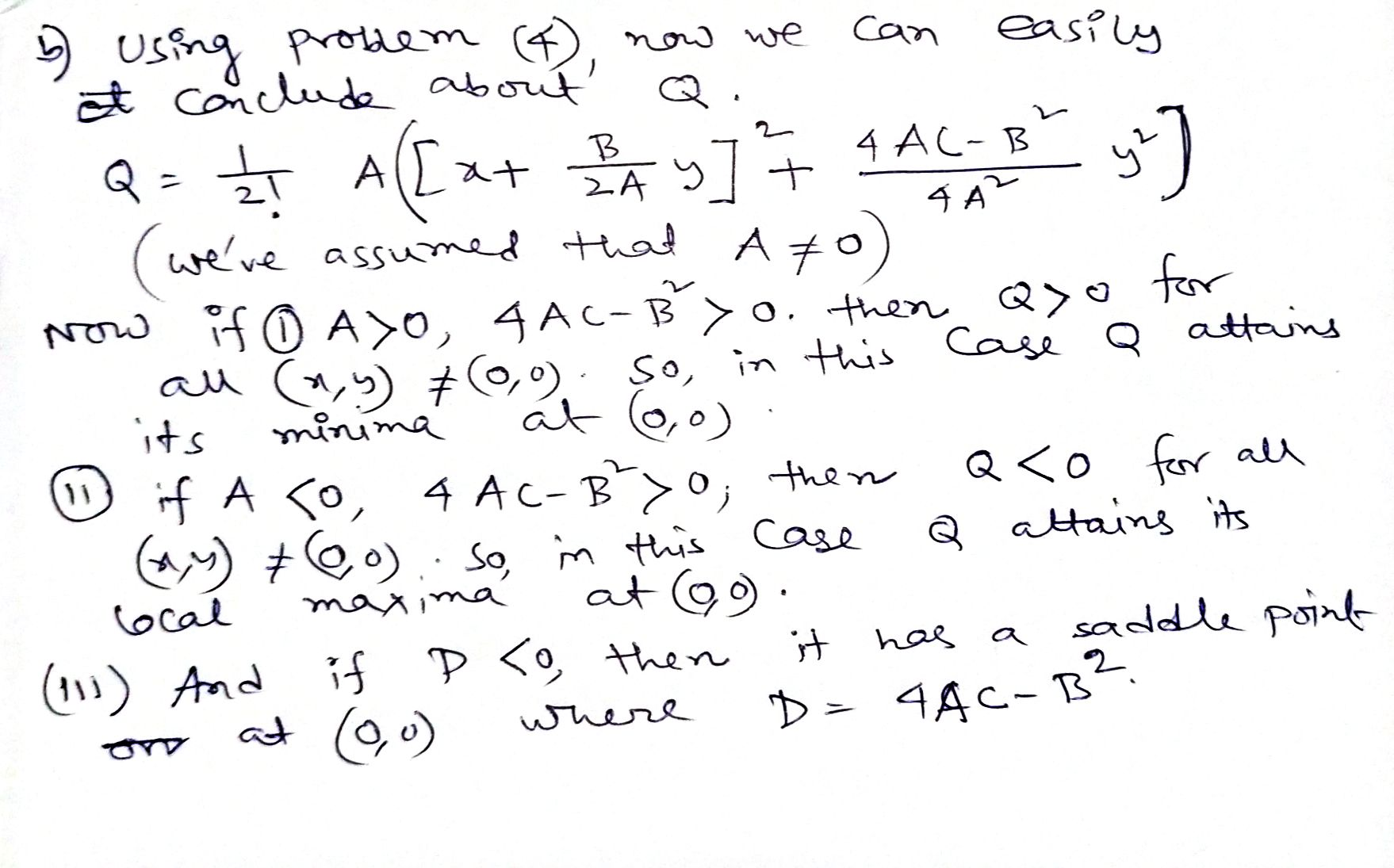##### Add Answer of: Please do question 5a and 5b 4. In this problem we analyze the behavior of the...
Similar Homework Help Questions
• ### Anlayze behavior of Polynomial

Let f(x,y)= ax2+bxy+y2 show by completing thesquare that : f(x,y)=ax2+bxy+y2=a[(x+((b/2a)*y))2+((4ac-b2)/4a2)y2)]and for D=4ac-b2 show: if D>0 and a<0 f has localminimum at (0,0), if D>0 and a<0 f has local maximum at (0,0),if D<0 then(0,0) is a saddlepoint.Thanks I ll rate with lifesaver

• ### Consider the polynomial f(x,y)=ax^2+bxy+cy^2 (without using second derivative test) by identifying the graph as a paraboloid. ***Graph at least 9 DIFFERENT polynomials. Show graphs to accompany ac...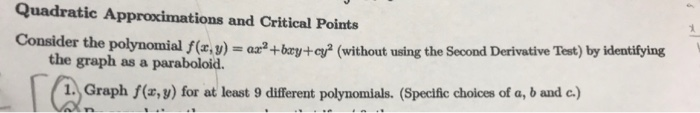Consider the polynomial f(x,y)=ax^2+bxy+cy^2 (without using second derivative test) by identifying the graph as a paraboloid. ***Graph at least 9 DIFFERENT polynomials. Show graphs to accompany actual working. Would appreciate it dearly. Quadratic Approximations and Critical Points Consider the polynomial f(x,y)+ ry+ c (without using the Second Derivative Tet) by identifying the graph as a paraboloid. 1. Graph f(x, y) for at least 9 different polynomials. (Specific choices of a, b and c.) Quadratic Approximations and Critical Points Consider the...

• ### (1 point) Consider the function defined by F(x, y) = x2 + y2 except at (r, y) - (0, 0) where F(0,0)0 Then we have (0,0)...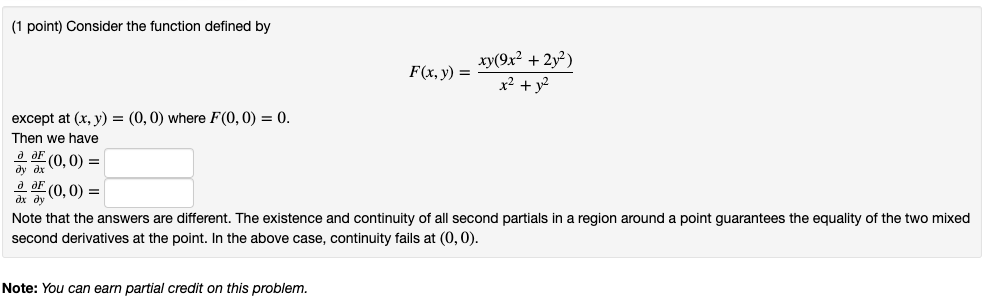(1 point) Consider the function defined by F(x, y) = x2 + y2 except at (r, y) - (0, 0) where F(0,0)0 Then we have (0,0) = (0,0) = ax dy Note that the answers are different. The existence and continuity of all second partials in a region around a point guarantees the equality of the two mixed second derivatives at the point. In the above case, continuity fails at (0,0) Note: You can earn partial credit on this problem...

• ### 3 This problem is to prove the following in the precise fashion described in class: Let O C R2 be...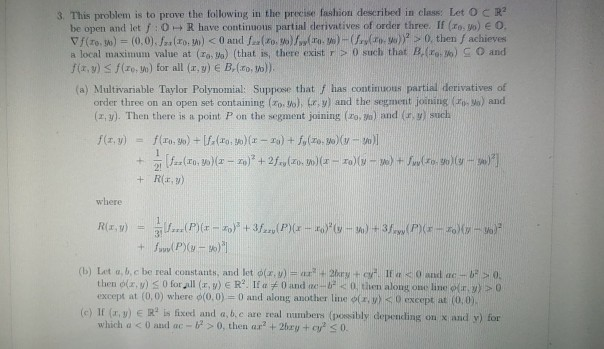Please write carefully! I just need part a and c done. Thank you. Will rate. 3 This problem is to prove the following in the precise fashion described in class: Let O C R2 be open and let f: 0+ R have continuous partial derivatives of order three. If (ro, o) O a local maximum value at (To, Va) (that is, there exist r > 0 such that B. (reo) O and (a) Multivariable Taylor Polynomial: Suppose that f has...

• ### 3. This problem is to prove the following in the precise fashion described in class: Let o sR be ...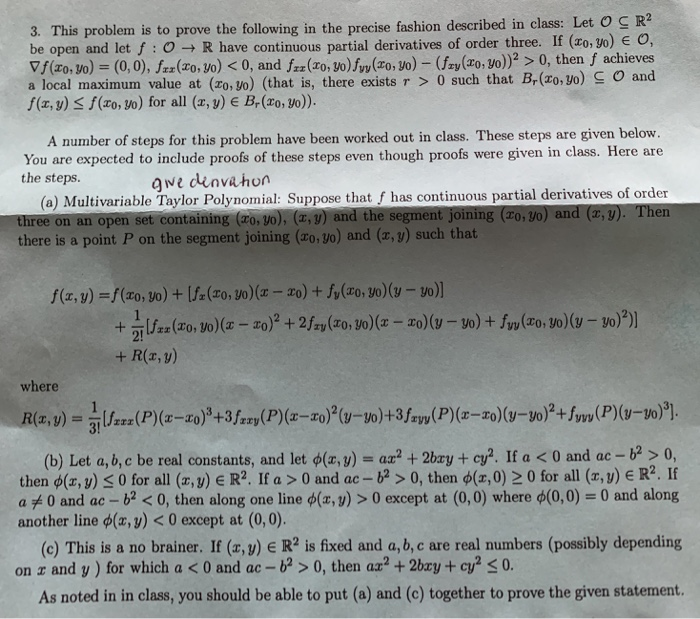3. This problem is to prove the following in the precise fashion described in class: Let o sR be open and let f :o, R have continuous partial derivatives of order three. If (o, 3o) ▽f(zo. ) = (0,0),Jar( , ) < 0, and fzz(z ,m)f (zo,yo) -(fe (a ,yo)) a local maximum value at (zo, yo) (that is, there exists r 0 such that B,(zo, yo) S O and f(a, y) 3 f(zo, yo) for all (x, y) e...

Previously I had to show that a quadratic form ax^2+bxy+cy^2 may be converted to a from AX^2+BY^2 by suitable choice of ? in the substitutionx=X cos?-Ysin?,y=X sin?+Y cos?,by checking that the coefficient of XY is (c-a)sin2?+bcos2?.I found that theata=1/2 tan^-1 (B/A-C). From this I deduced that by suitable rotation of axes, any quadratic curve may be expressed in the formAX^2+BY^2+CX+DY+E=0If both A and B are nonzero in the equation from the second problem, show that a shift of origin (a...

• ### if you know the answer for all parts then do it otherwise skip it please If...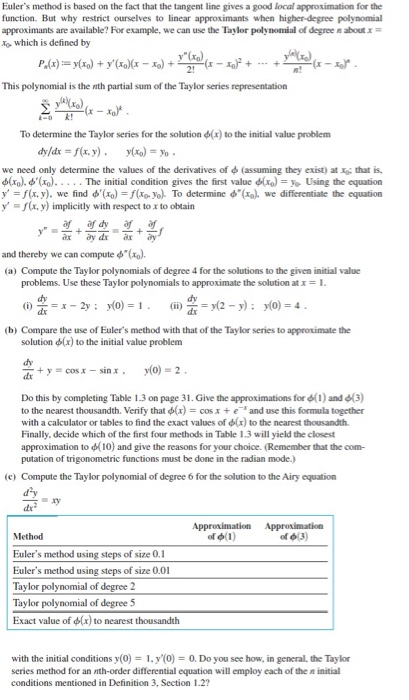if you know the answer for all parts then do it otherwise skip it please If iam not satisfied with ur answer i will give 5 dislikes from me and my friends Answer should be clear and good handwriting If you don’t know the correct answer its better to skip Dont copy and paste from previous chegg answers Euler's method is based on the fact that the tangent line gives a good local approximation for the function. But why restrict...

• ### 3. This problem is to prove the foll owing in the precise fashion described in class: Let O R2 eo...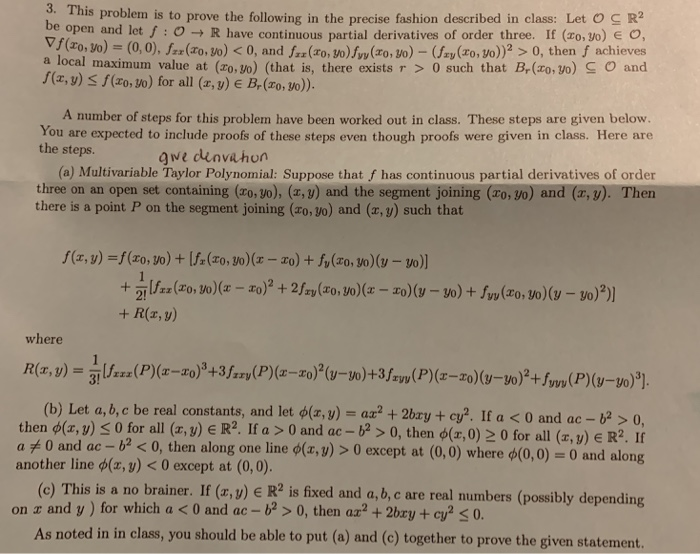3. This problem is to prove the foll owing in the precise fashion described in class: Let O R2 eopen and let/ : O → R have continuous partial derivatives of order three. If (zo,to) e o, )(0,0), fxr(ro, vo) < 0, and frr(ro, o)(ro, o)- ay(ro, Vo) 0, then f achieves a local maximum value at (zo. 5o) (that is, there exists 0 such that Br(o, vo) S O and (x, y) S f(xo, so) for all (x, y)...

• ### dont ans this question Euler's method is based on the fact that the tangent line gives...dont ans this question Euler's method is based on the fact that the tangent line gives a good local approximation for the function. But why restrict ourselves to linear approximants when higher degree polynomial approximants are available? For example, we can use the Taylor polynomial of degree about = No, which is defined by P.(x) = y(x) + y (xo)(x – Xa) + 21 (x- This polynomial is the nth partial sum of the Taylor series representation (te) (x –...

• ### Question 5 1, 19: + 'fax + Son + 4x + xy + yafya + xzbu...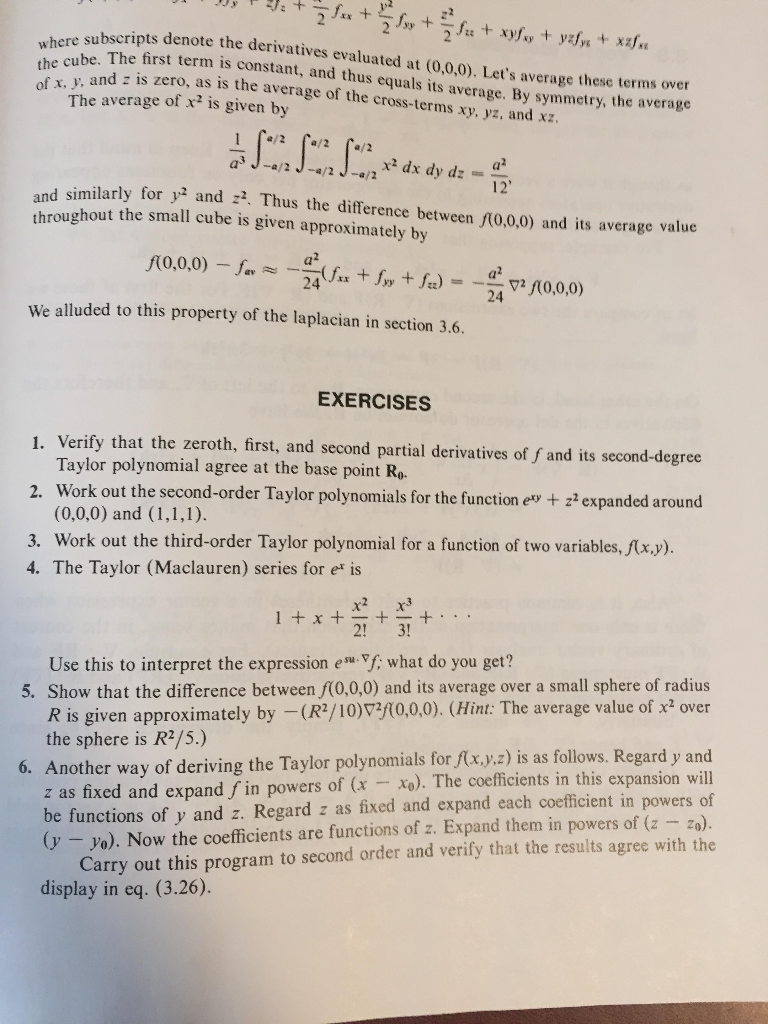Question 5 1, 19: + 'fax + Son + 4x + xy + yafya + xzbu where subscripts denote the cube. The first te of x, y, and z is zero, as is th ubscripts denote the derivatives evaluated at (0.0.0). Let's average these terms over . The first term is constant, and thus equals its average. By symmetry, the average d is zero, as is the average of the cross-terms xy, yz, and xz. The average of x- is...

Free Homework App